NCERT Questions

Class 10
Chapter 10 Class 10 - Light - Reflection and Refraction

## An object 5.0 cm in length is placed at a distance of 20 cm in front of a convex mirror of radius of curvature 30 cm. Find the position of the image, its nature and size.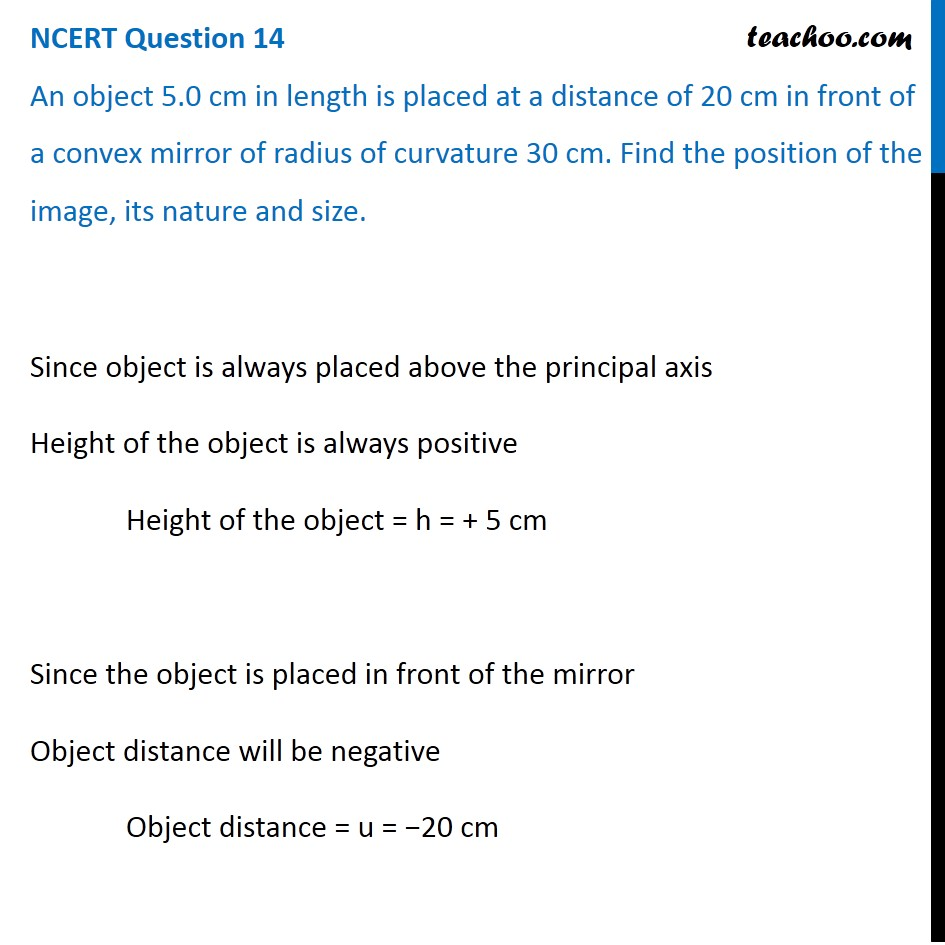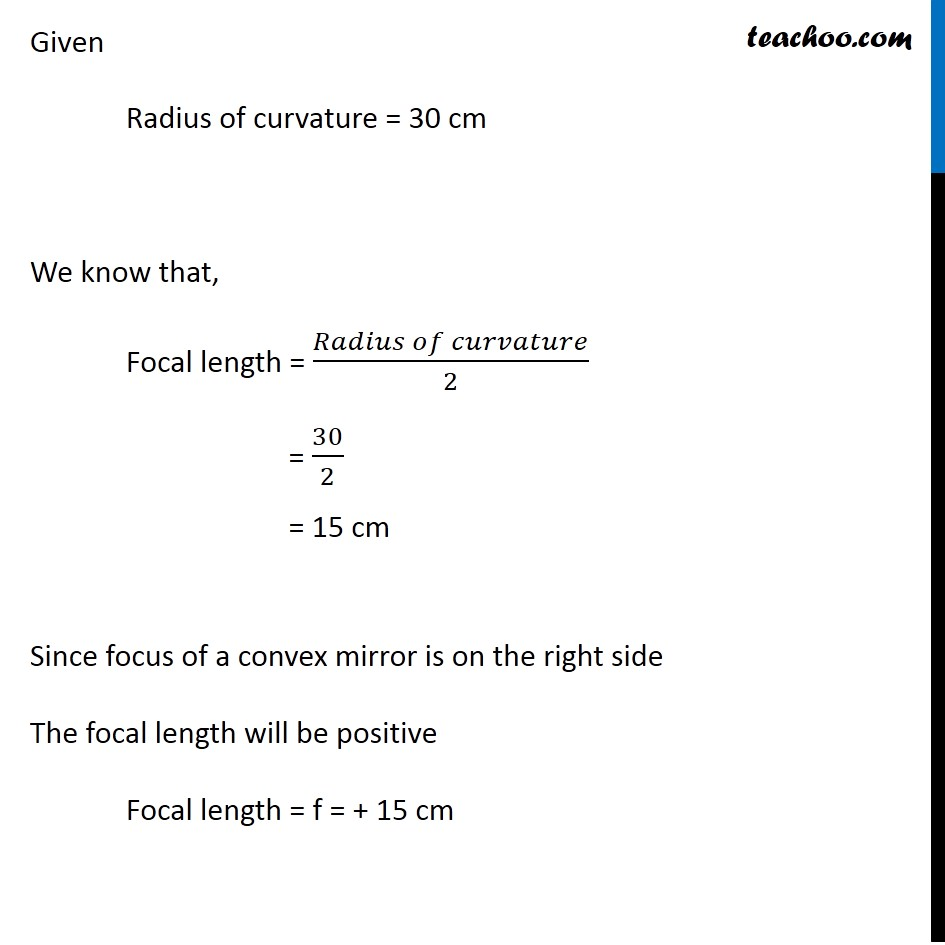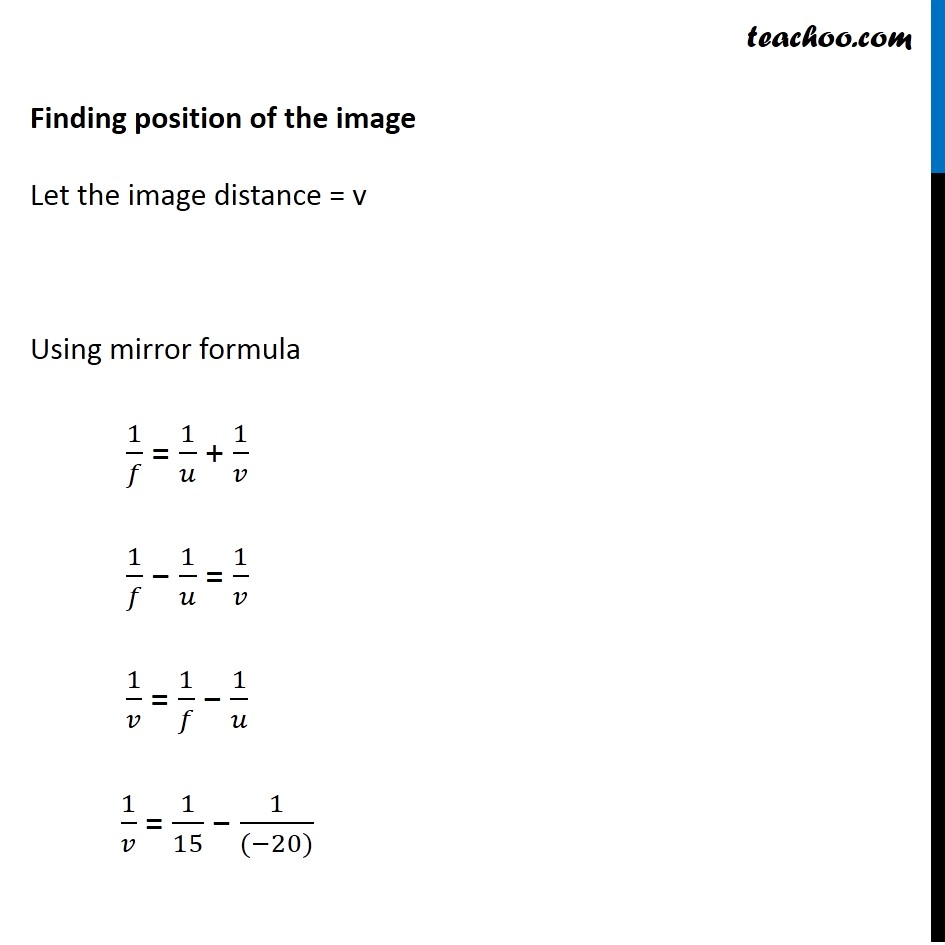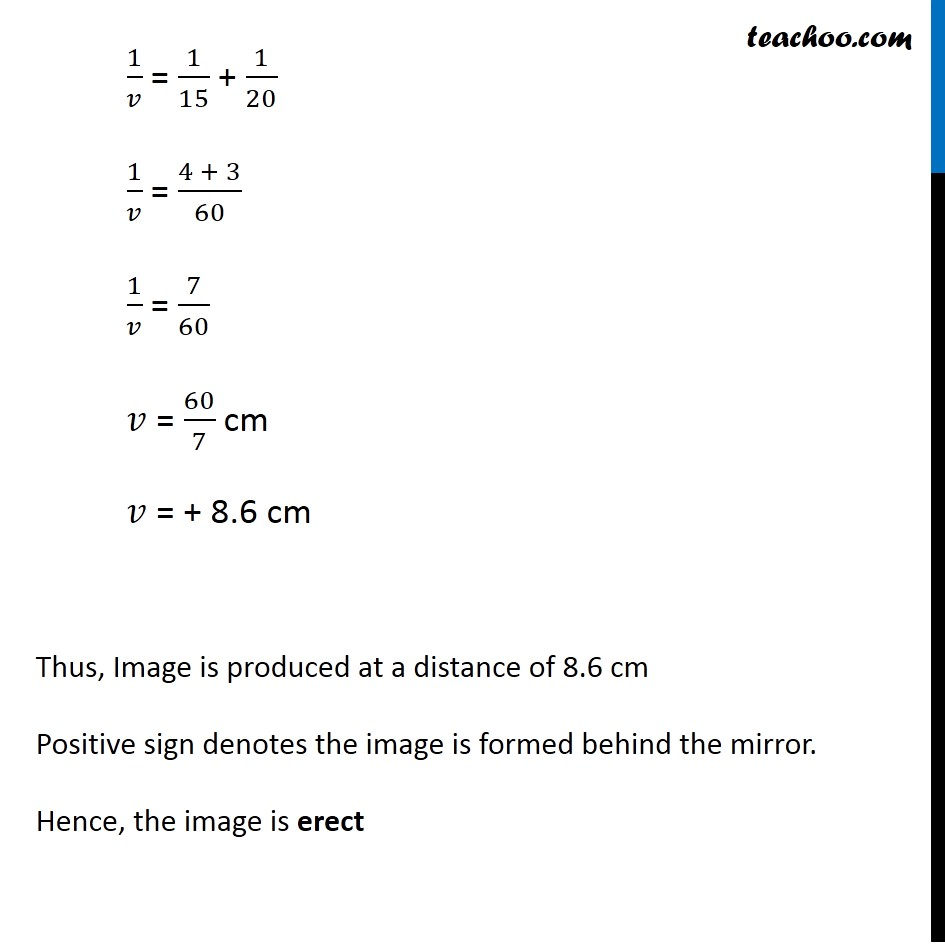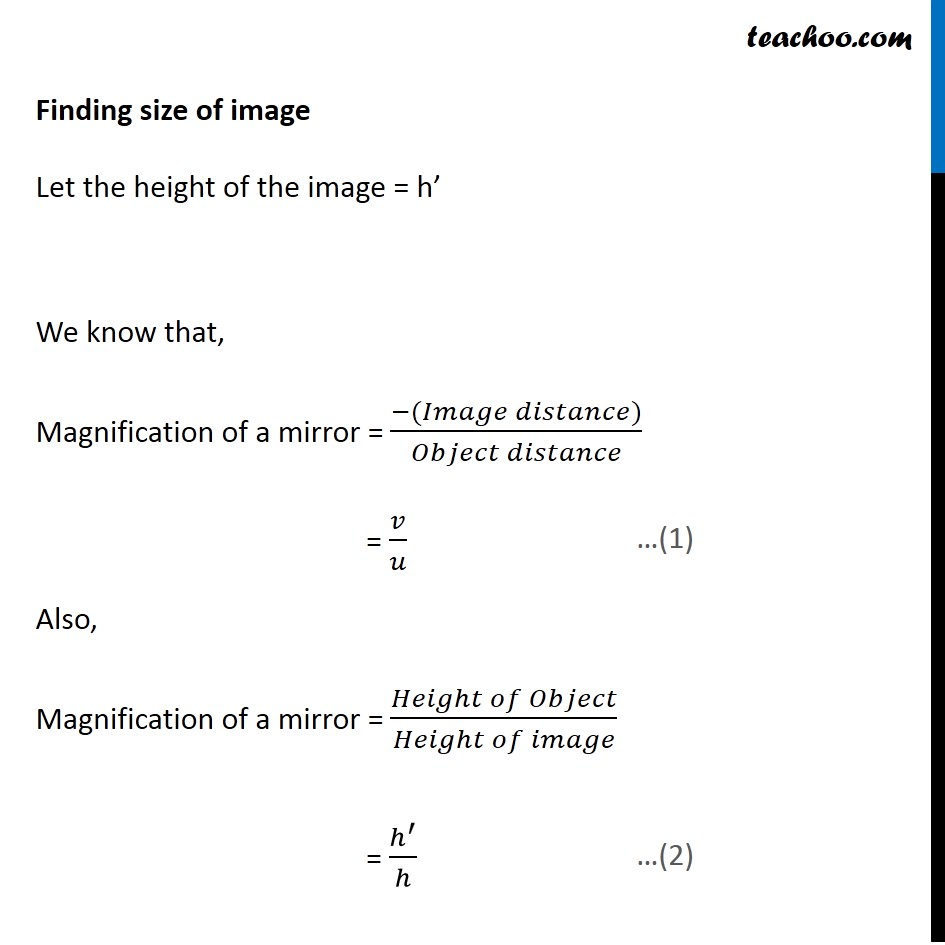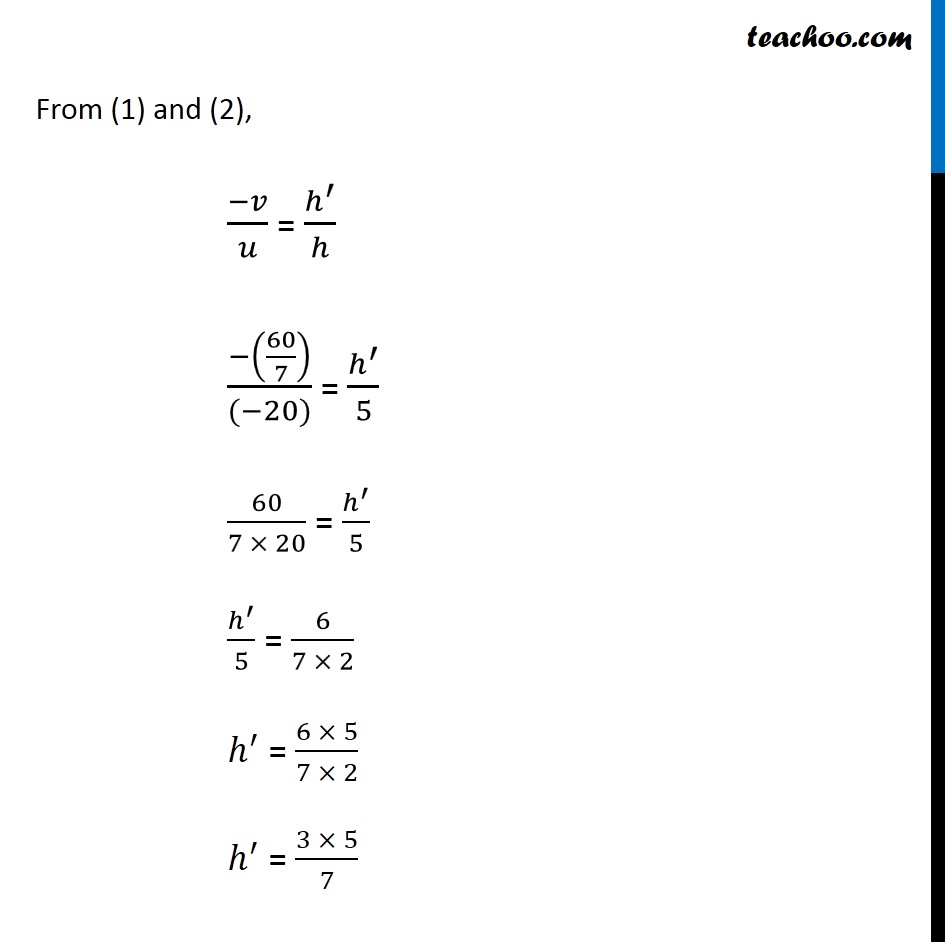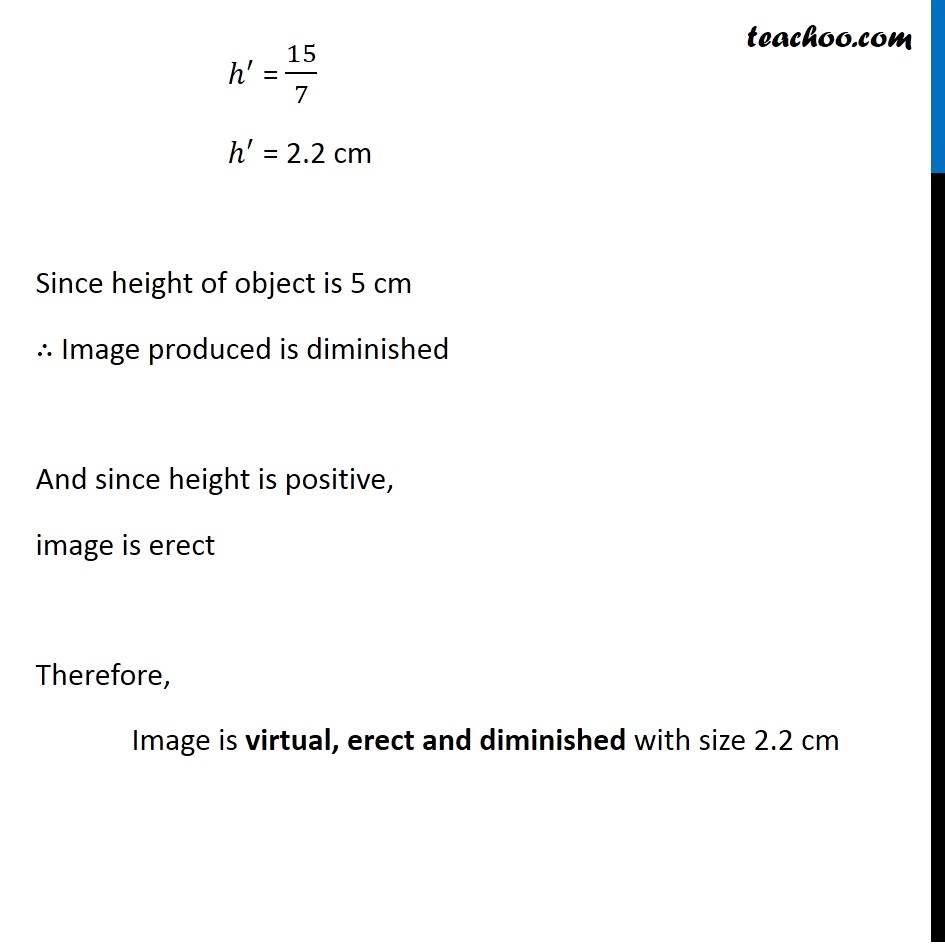Learn in your speed, with individual attention - Teachoo Maths 1-on-1 Class

### Transcript

NCERT Question 14 An object 5.0 cm in length is placed at a distance of 20 cm in front of a convex mirror of radius of curvature 30 cm. Find the position of the image, its nature and size. Since object is always placed above the principal axis Height of the object is always positive Height of the object = h = + 5 cm Since the object is placed in front of the mirror Object distance will be negative Object distance = u = −20 cm Given Radius of curvature = 30 cm We know that, Focal length = (𝑅𝑎𝑑𝑖𝑢𝑠 𝑜𝑓 𝑐𝑢𝑟𝑣𝑎𝑡𝑢𝑟𝑒)/2 = 30/2 = 15 cm Since focus of a convex mirror is on the right side The focal length will be positive Focal length = f = + 15 cm Finding position of the image Let the image distance = v Using mirror formula 1/𝑓 = 1/𝑢 + 1/𝑣 1/𝑓 − 1/𝑢 = 1/𝑣 1/𝑣 = 1/𝑓 − 1/𝑢 1/𝑣 = 1/15 − 1/((−20)) 1/𝑣 = 1/15 + 1/20 1/𝑣 = (4 + 3)/60 1/𝑣 = 7/60 𝑣 = 60/7 cm 𝑣 = + 8.6 cm Thus, Image is produced at a distance of 8.6 cm Positive sign denotes the image is formed behind the mirror. Hence, the image is erect Finding size of image Let the height of the image = h’ We know that, Magnification of a mirror = (−(𝐼𝑚𝑎𝑔𝑒 𝑑𝑖𝑠𝑡𝑎𝑛𝑐𝑒))/(𝑂𝑏𝑗𝑒𝑐𝑡 𝑑𝑖𝑠𝑡𝑎𝑛𝑐𝑒) = 𝑣/𝑢 Also, Magnification of a mirror = (𝐻𝑒𝑖𝑔ℎ𝑡 𝑜𝑓 𝑂𝑏𝑗𝑒𝑐𝑡)/(𝐻𝑒𝑖𝑔ℎ𝑡 𝑜𝑓 𝑖𝑚𝑎𝑔𝑒) = ℎ^′/ℎ From (1) and (2), (−𝑣)/𝑢 = ℎ^′/ℎ (−(60/7))/((−20)) = ℎ^′/5 60/(7 × 20) = ℎ^′/5 ℎ^′/5 = 6/(7 × 2) ℎ^′ = (6 × 5)/(7 × 2) ℎ^′ = (3 × 5)/7 ℎ^′ = 15/7 ℎ^′ = 2.2 cm Since height of object is 5 cm ∴ Image produced is diminished And since height is positive, image is erect Therefore, Image is virtual, erect and diminished with size 2.2 cm